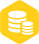# FIR数字滤波器设计

### 描述

FIR数字滤波器设计（上）

FIR数字滤波器设计（中）

FIR数字滤波器设计

FIR数字滤波器的设计方法有窗函数法、频率采样法和基于firls函数和remez函数的最优化方法。MATLAB语言中的数字信号处理工具箱，提供了一些滤波器的函数，使FIR滤波器的运算更加方便和快捷。

Firls()和remez()的基本格式用于设计I型和II型线性相位FIR滤波器，I型和II型的区别是偶函数还是奇函数。freqz()用于求数字滤波器的频率响应。并且提供了各种窗函数的函数，比如，hamming()是海明窗函数，hanning()是汉宁窗函数，kaiser()是凯泽窗函数，使在设计的过程中，不用自己重新设计窗函数。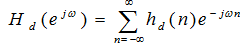（3-1）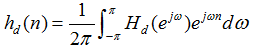（3-2)（3-3）

w(n)是有限长序列，当n<0或n>N-1时，w(n)=0。

1、矩形窗(Rectangle Window)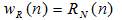（3-4）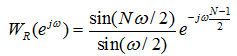（3-5）

2、三角形窗(Bartlett Window)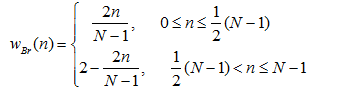（3-6）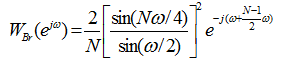（3-7)

3、汉宁(Hanning)窗，又称升余弦窗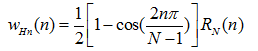（3-8）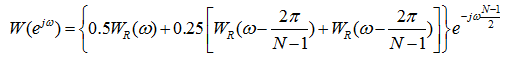（3-9）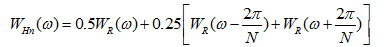(3-10)

4、汉明(Hamming)窗，又称改进的升余弦窗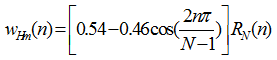（3-11）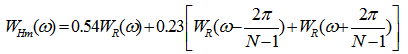（3-12）

5、布莱克曼(Blankman)窗，又称二阶升余弦窗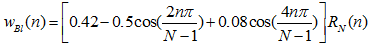（3-13)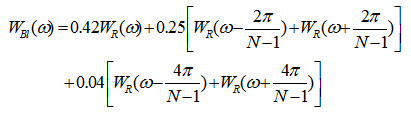(3-14）

6、凯泽(Kaiser)窗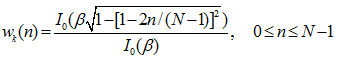（3-15）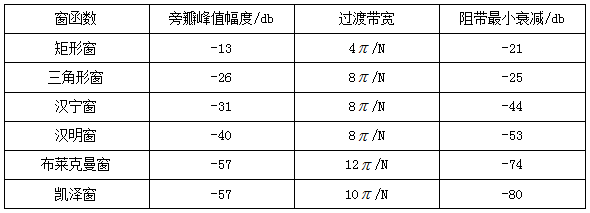1）根据技术要求确定等待求值滤波器的单位取样响应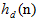2）根据对过渡带和阻带衰减的要求，选择窗函数的形式，并估计窗口长度N。

3）计算滤波器的单位取样响应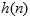（3-16)

4）检验技术指标是否满足要求。根据下式计算：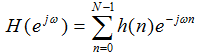(3-17)

1、程序如下：

Wp=0.4*pi;Ws=0.5*pi;Wdel=Ws-Wp;N=ceil(8*pi/Wdel);Wn=(0.4+0.5)*pi/2;window=hanning(N+1);b=fir1(N,Wn/pi,window);freqz(b,1,512)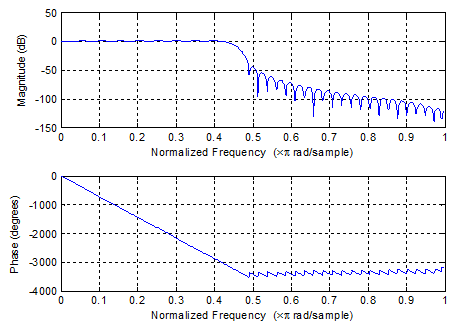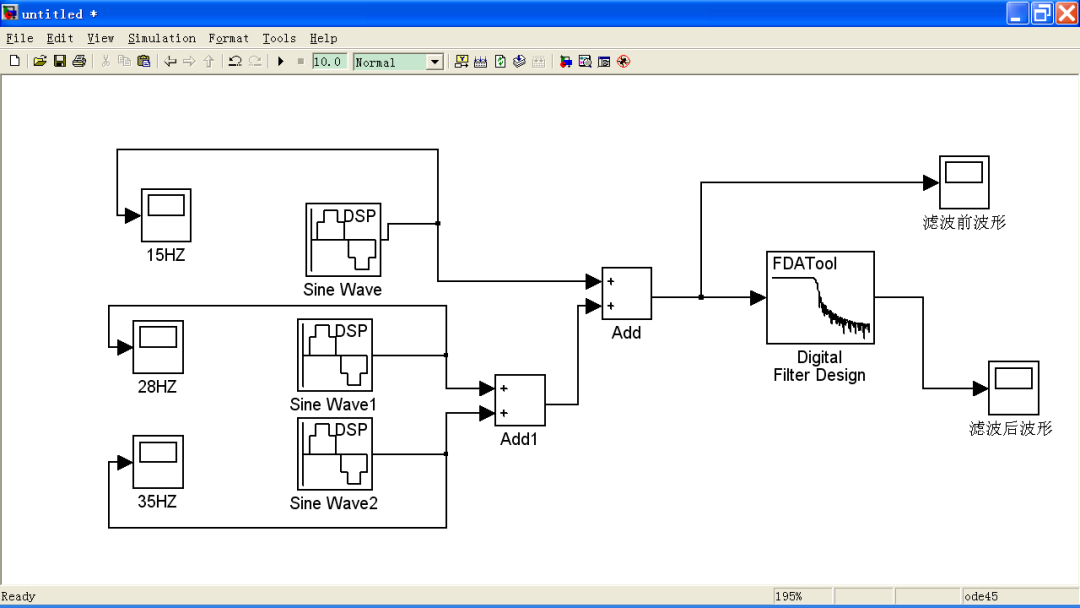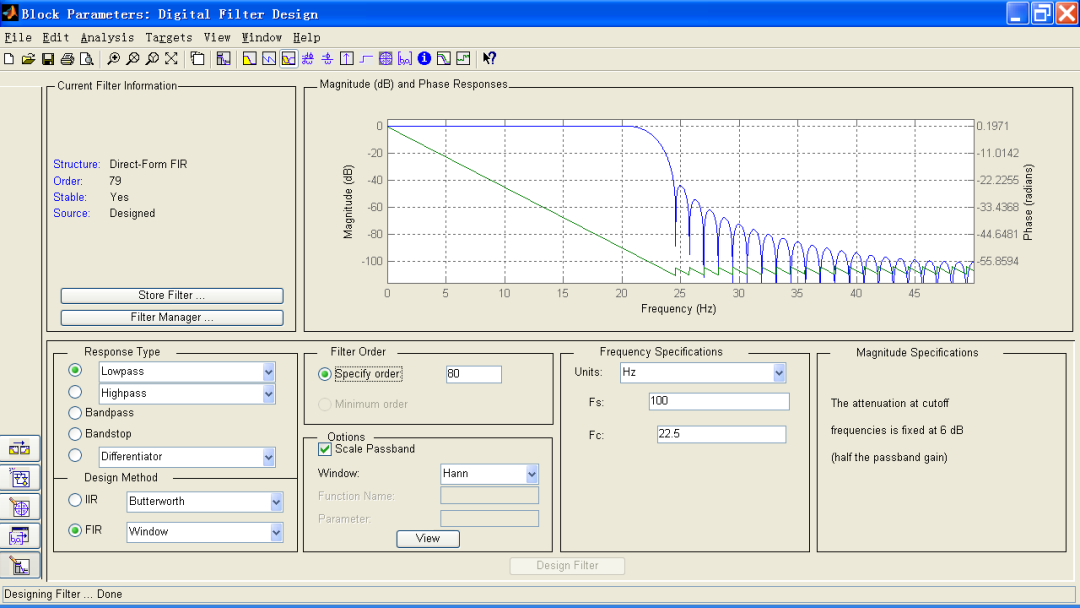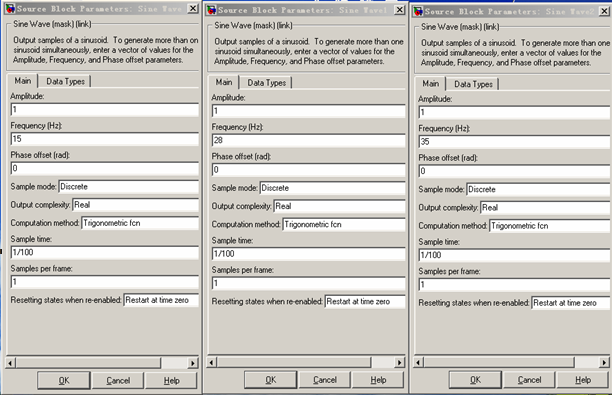15HZ信号波形如下：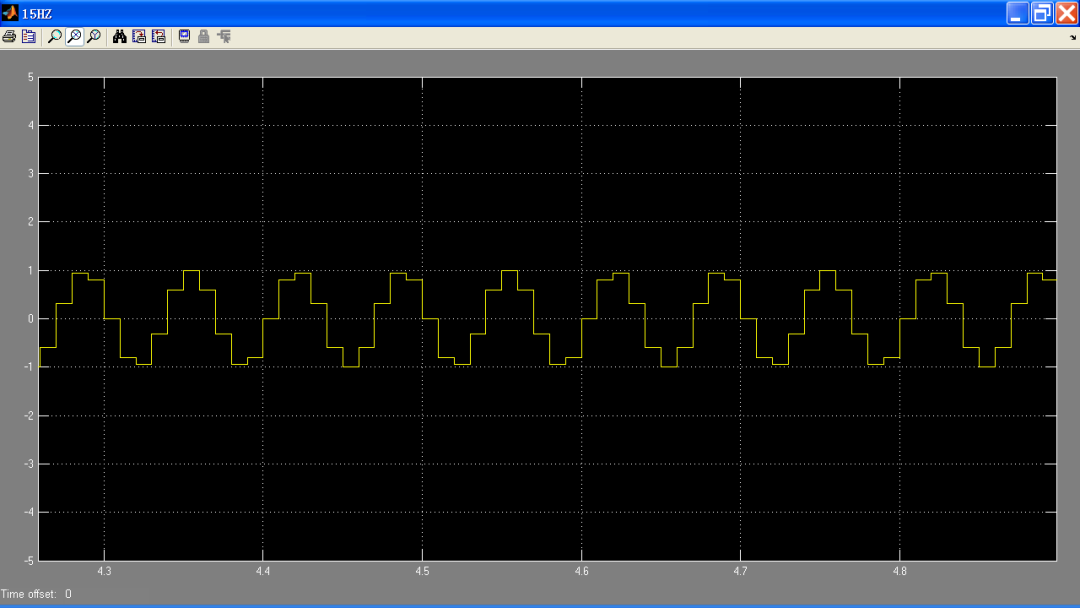28HZ信号波形如下：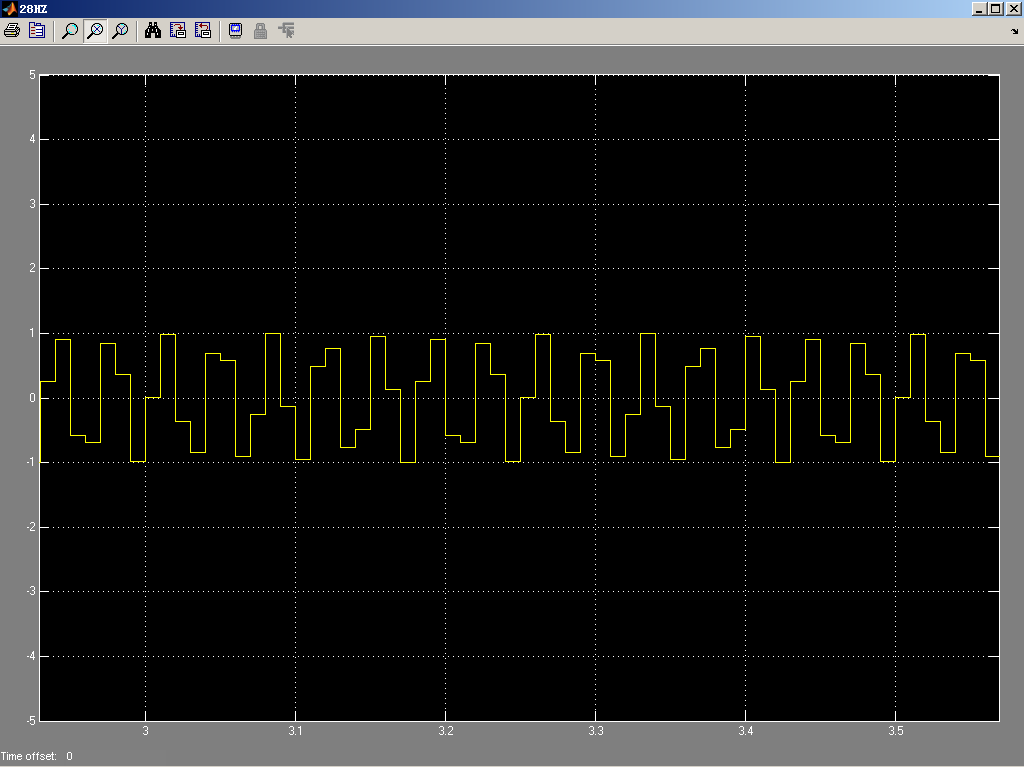35HZ信号波形如下：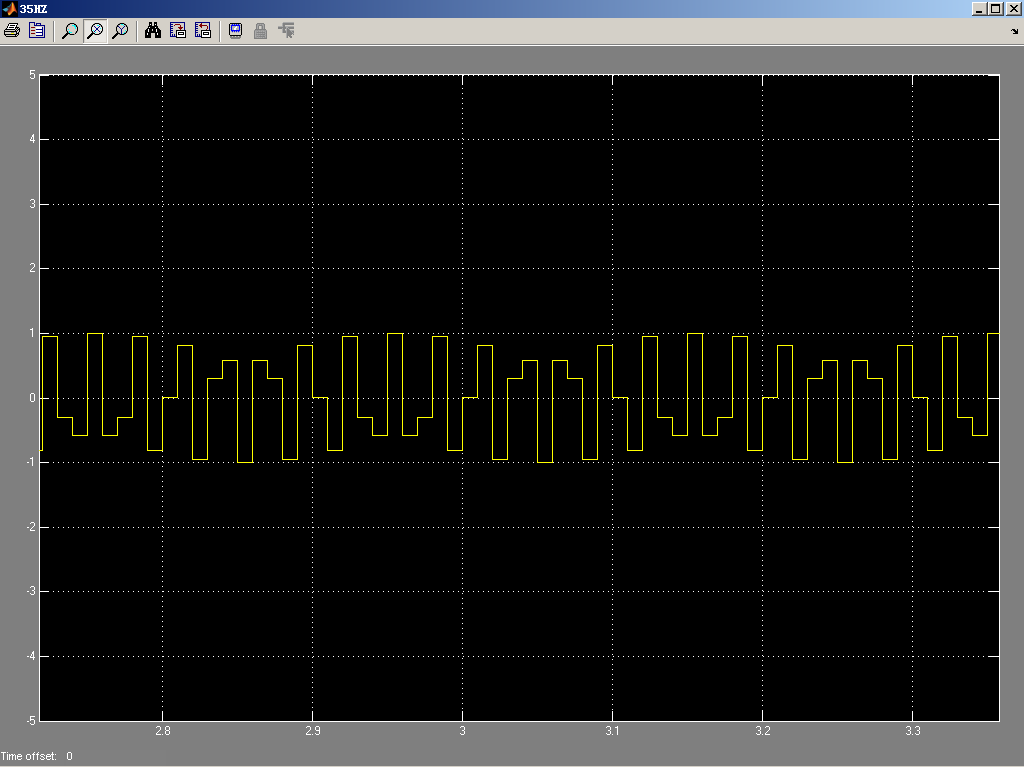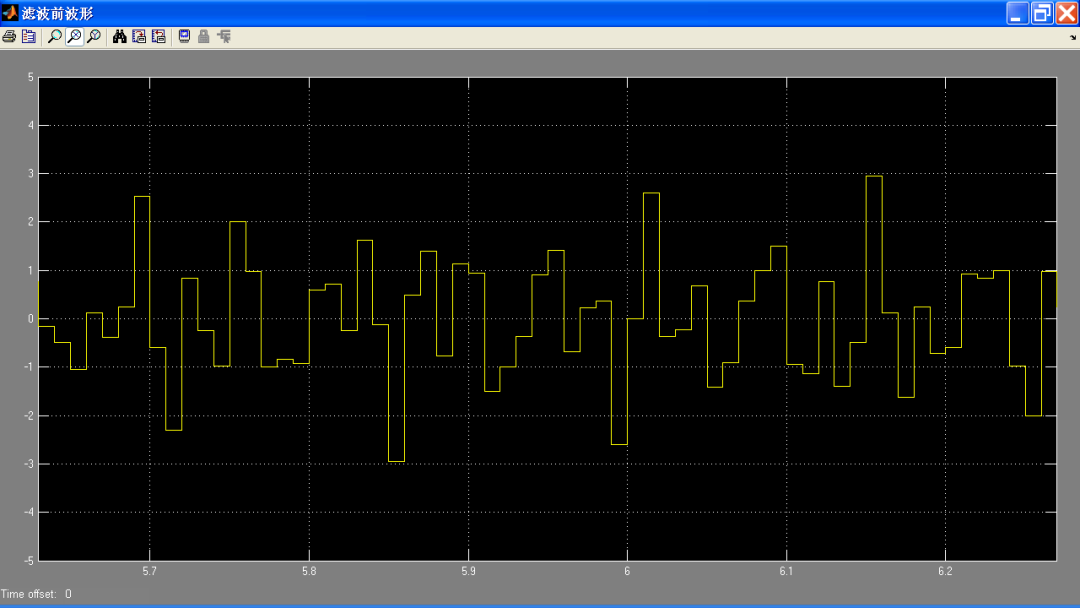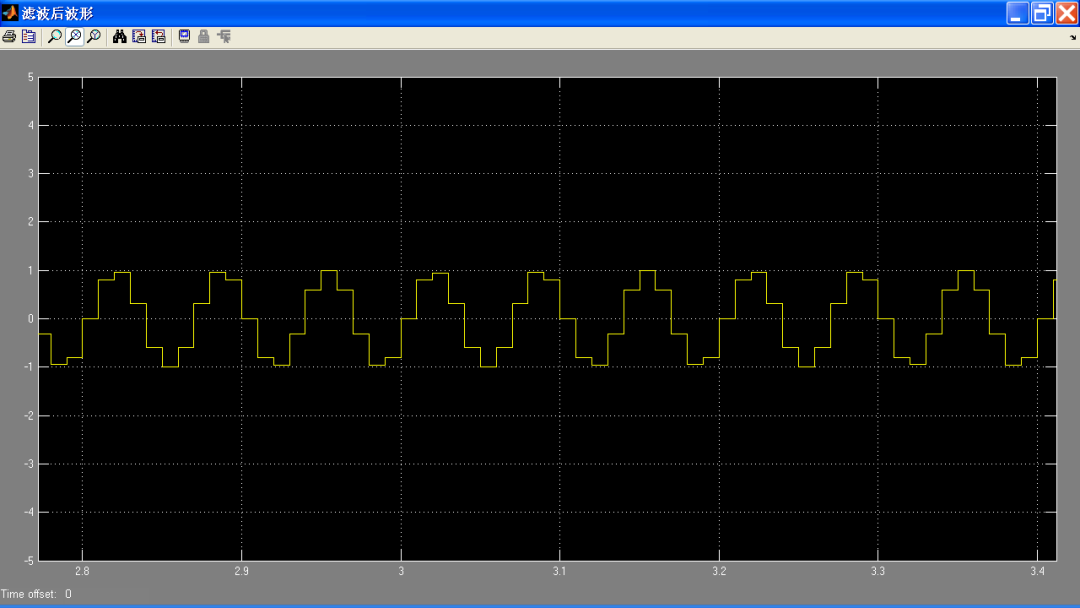1、对理想滤波器的系统函数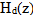进行频率采样以得到系统的理想频响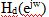的等间隔采样值H(k)。

H(k)实际上是所要求的滤波器的单位采样响应(h(n))的离散傅里叶变换（DFT），如下关系式：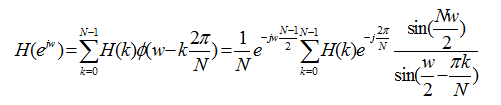(3-18)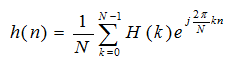(3-19)

(1)给定理想滤波器频率响应(2)根据过渡带宽和阻带衰减确定过渡点数和h(n)的长度N。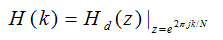(3-20)

(3)由IFFT计算IDFT得到：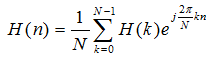(3-21)

M=40;al=(M-1)/2;l=0:M-1;t1=0.109021;t2=0.59417456;Hrs=[zeros(1,5),t1,t2,ones(1,7),t2,t1,zeros(1,9),t1,t2,ones(1,7),t2,t1,zeros(1,4)];k1=0:floor((M-1)/2);k2=floor((M-1)/2)+1:M-1;angh=[-al*(2*pi)/M*k1,al*(2*pi)/M*(M-k2)];H=Hrs.*exp(j*angh);h=real(ifft(H,M));freqz(h,1,512,1000)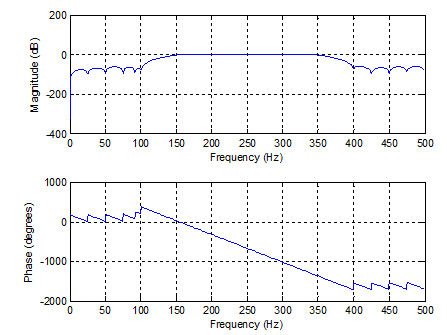MATLAB信号处理工具箱提供了通用的工具函数firls和remez，采用不同的优化方法可以设计最优滤波器和多频带滤波器。

firls是fir1和fir2函数的扩展，其基本设计准则是利用最小二乘法使期望的频率响应和实际的频率响应之间的整体误差最小。

Remez函数则利用Parks-McClellan算法设计线性相位FIR滤波器。

1） firls。

b=firls(n,f,a)

b=firls(n,f,a,w)

b=firls(n,f,a,’ftype’)

b=firls(n,f,a,w,’ftype’)

b=firls(n,f,a,w)则使用权系数w给误差加权。

b=firls(n,f,a,w,’ftype’)调用形式中，参数’ftype’用于指定所设计的滤波器类型，ftype=Hilbert，为奇对称的线性相位滤波器。

2） 函数remez的基本调用格式为：b=remez(n,f,a)。

1、程序如下所示：

f=[0 0.3 0.4 0.6 0.7 1];m=[0 0 1 1 0 0];n=20;b=firls(n,f,m); %firls函数设计FIR数字滤波器[h,w]=freqz(b); bb=remez(n,f,m); %remez函数设计FIR数字滤波器[hh,w]=freqz(bb);axes('position',[0.2 0.2 0.5 0.5]); plot(w/pi,abs(h),'b:',w/pi,abs(hh),'r-');xlabel('frequency');ylabel('magnitude');grid on;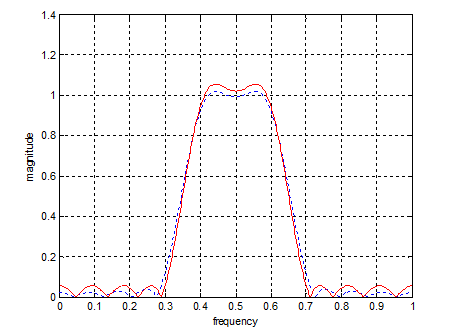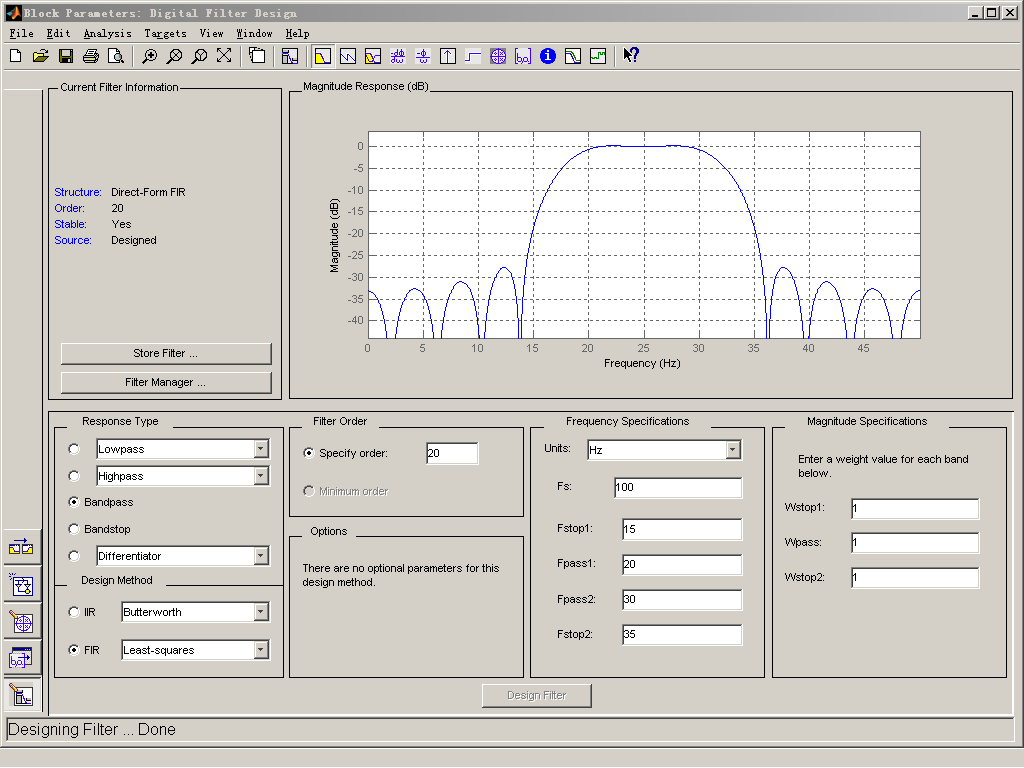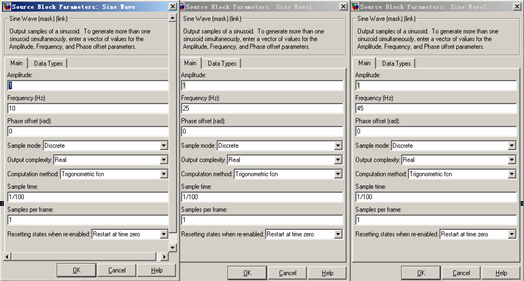（1）10HZ信号波形如下所示：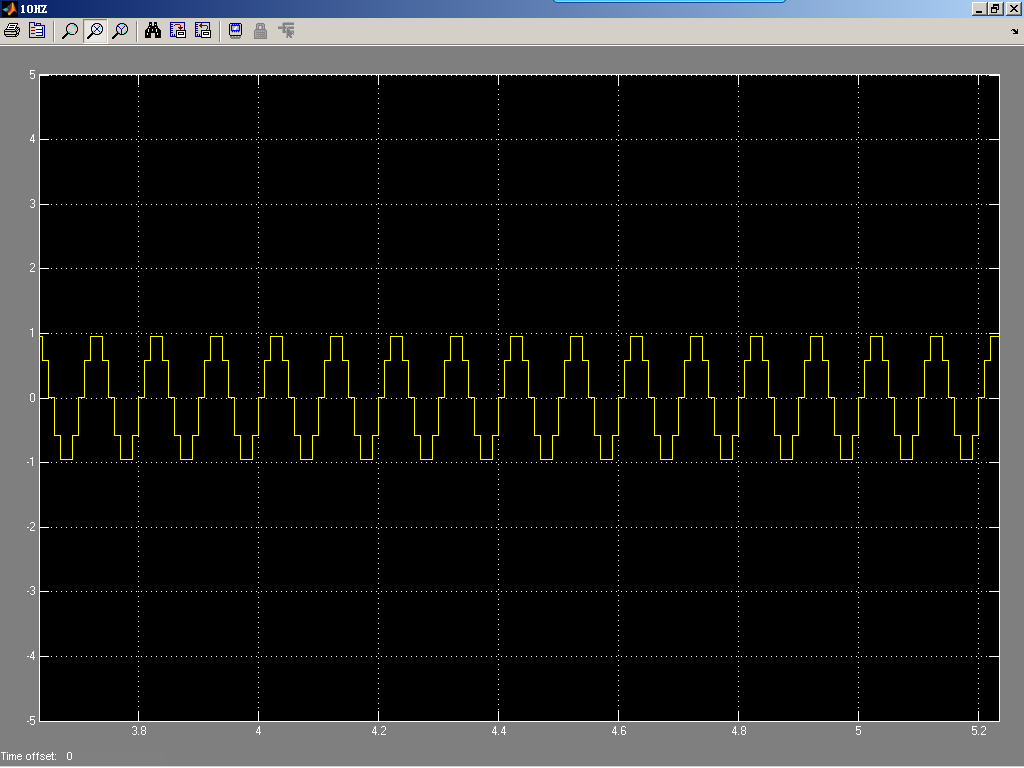（2） 25HZ信号波形如下所示：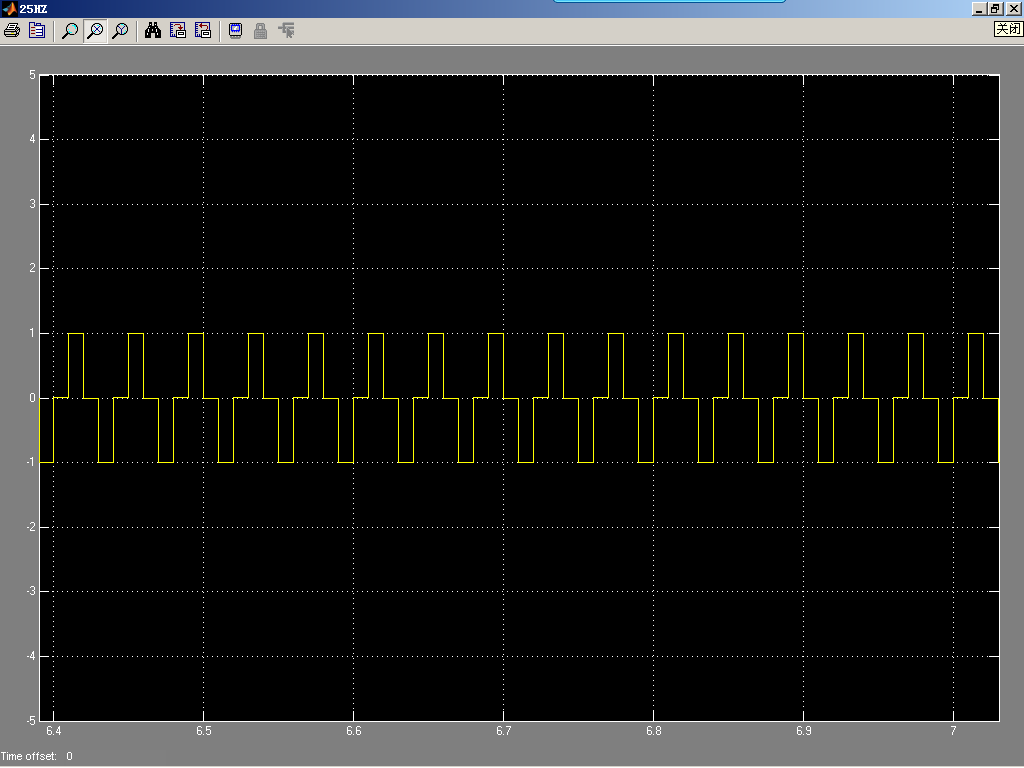（3）45HZ信号波形如下所示：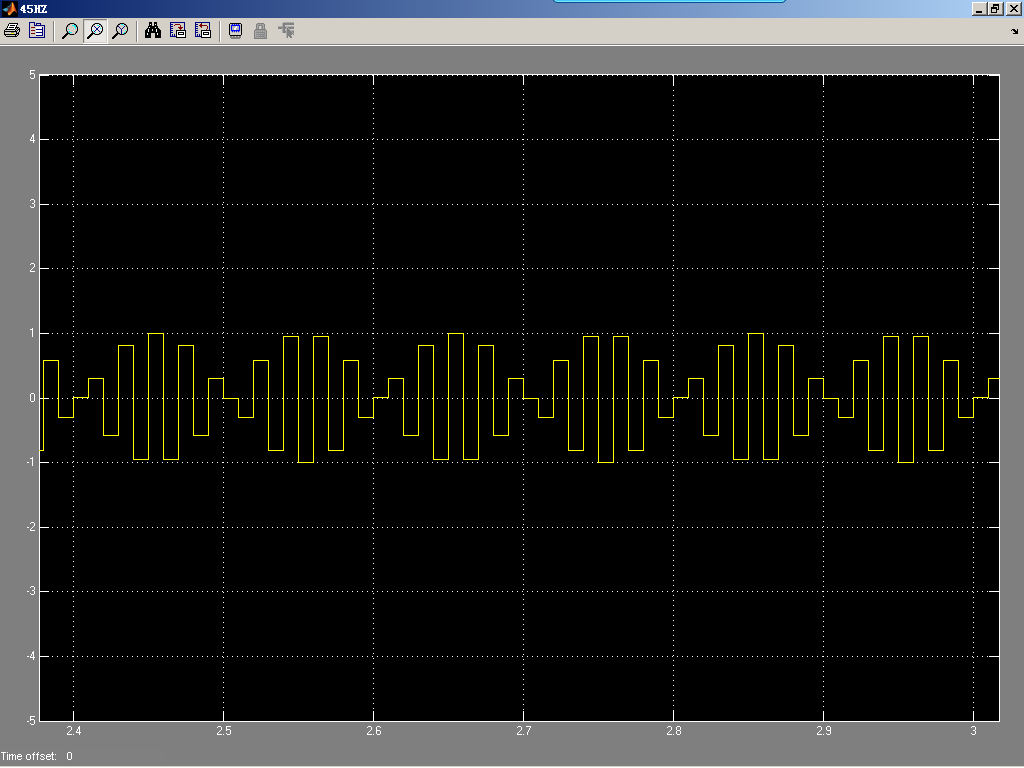（4）滤波前波形如下所示：（5）滤波后波形如下所示：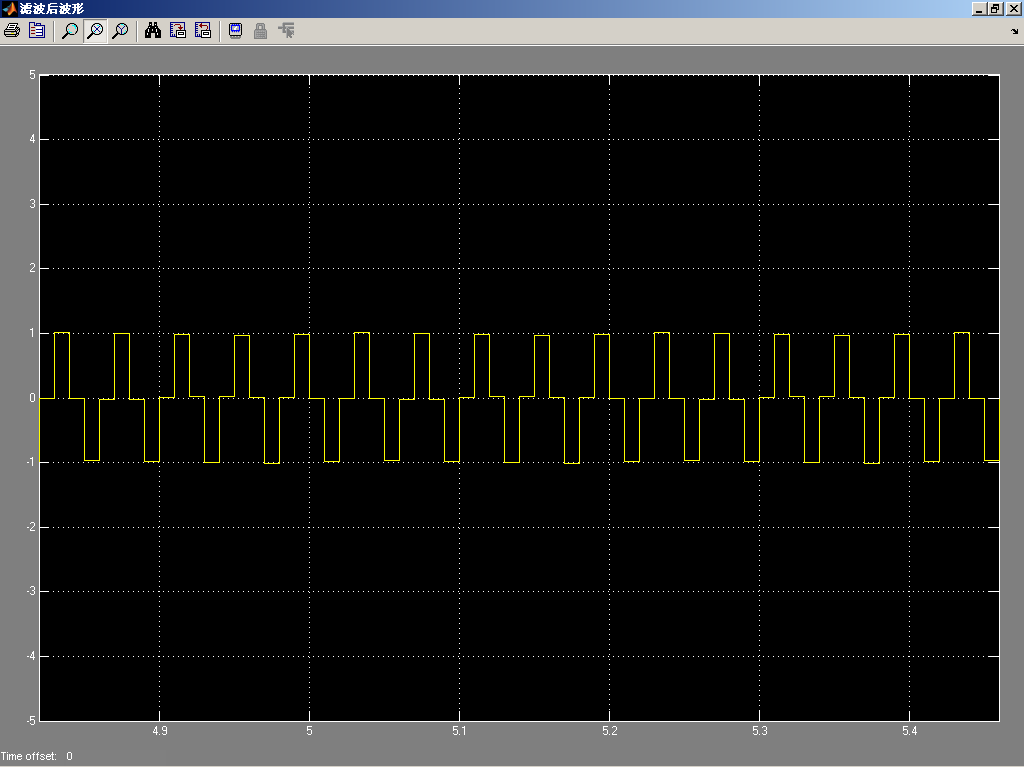×
20